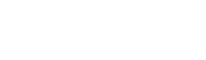# 【LPIPS・SSIM・PSNR・EMD】Summary of image similarity indicators

スポンサーリンク

Hello, I am Yuuki (@engineerblog_Yu), who studies visualization in the simulation laboratory.

I would like to summarize the image similarity index used to evaluate images generated using GAN.

スポンサーリンク

## PSNR(Peak Signal to NoizeRatio)

PSNR calculates the ratio of maximum pixel value to noise.

$$PSNR=10log_{10}\frac{MAX^2}{MSE}$$

(MAX: maximum pixel value, MSE: mean squared error)

With PSNR, however, (considerably different locally) ≈ (slightly different overall), and no difference can be detected.

PSNR is not a good match for human vision, because human visual characteristics would perceive a slight overall difference to be less similar.

## SSIM(Structual Similarity)

SSIM can evaluate changes in pixel value (luminance), contrast, and structure.

SSIM is an index designed to improve the problems of PSNR and is defined by the following formula

$$SSIM(x,y)=\frac{(2μ_xμ_y+C_1)(2σ_{xy}+C_2)}{(μ^2_{x}+μ^2_y+C_1)(σ^2_x+σ^2_y+C_2)}$$

(C: constant, σ: standard deviation, μ: average pixel value)

## EMD(Earth Mover’s Distance)

EMD is a distance measure, such as Euclidean distance, that measures the distance between the distributions of pixel values in an image.

If the distribution of pixel values in the images are similar, the images can be considered highly similar.

## LPIPS(Learned Perceptual Image Patch Similarity)

LPIPS is a criterion based on the features output by the convolutional layer of trained image classification networks such as AlexNet and VGG.

The official paper on LPIPS can be found at bellow.

The Unreasonable Effectiveness of Deep Features as a Perceptual Metric

As can be seen from the above image on the official page, Humans (human senses) and indicators such as PSNR and SSIM do not match well.

In contrast, indicators using trained networks often seem to correctly capture human senses.

Since SSIM and PSNR can only focus on pixel luminance and contrast, LPIPS, which is based on features, is said to be generally more accurate by neural networks.

## At the End

In this article, we briefly summarize SSIM, PSNR, EMD, and LPIPS used as image similarity measures.

LPIPS is said to be the most accurate, but we often see SSIM, PSNR, and EMD used as error evaluation indices in the latest papers.

It will be interesting to see if better image similarity indices will be developed in the future.

スポンサーリンク
Yukiをフォローするautotech
タイトルとURLをコピーしました